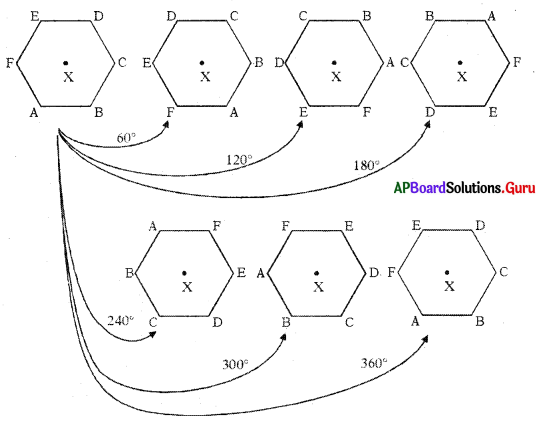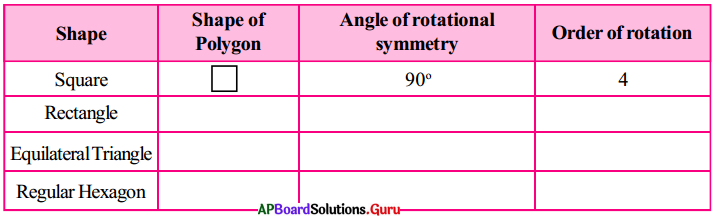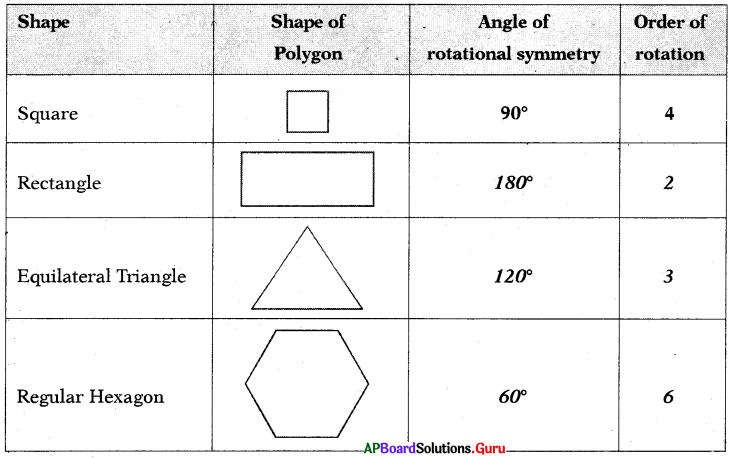# AP Board 7th Class Maths Solutions Chapter 12 Symmetry Ex 12.2

SCERT AP 7th Class Maths Solutions Pdf Chapter 12 Symmetry Ex 12.2 Textbook Exercise Questions and Answers.

## AP State Syllabus 7th Class Maths Solutions 12th Lesson Symmetry Ex 12.2

Question 1.
Which of the following shapes have line symmetry? Which have rotational symmetry?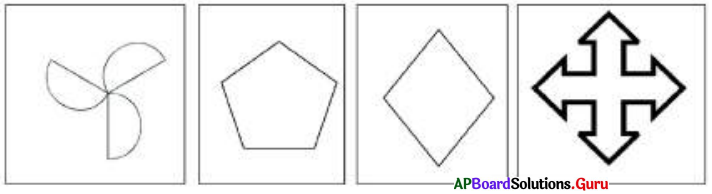(a) Has no line of symmetry and rotational symmetry.
(b) Has line of symmetry and no rotational symmetry.
(c) Has line of symmetry and rotational symmetry.
(d) Has line of symmetry and rotational symmetry.Question 2.
Determine the order of rotational symmetry in the figures given below.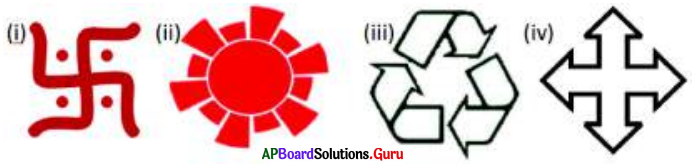(i) The order of rotatiorlal symmetry of the figure is 4.
(ii) The order of rotational symmetry of the figure is 6.
(iii) The order of rotational symmetry of the figure is 3.
(iv) The order of rotational symmetry of the figure is 4.

Question 3.
Draw the 2 such figures which have line symmetry and rotational symmetry of order more than 1.
(a) Equilateral triangle has 3 lines of symmetry and rotational symmetry of order (3).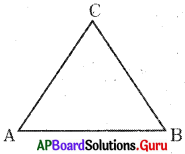(b) Rectangle has 2 lines of symmetry and rotational symmetry of order (2).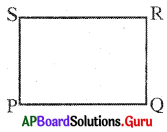Question 4.
Name the quadrilaterals which have both line symmetry and rotational symmetry of order more than 1.
Rectangle, square and rhombus.

Question 5.
After rotating by 60° about its axis, a figure looks exactly the same as its original position. What other angles will this happen for the same figure?